:

# EAN 13 BARCODE

## Format of the EAN 13

Length Type checksum
13 digits numeric yes

The EAN 13 is composed by 12 data digits + 1 checksum digit.

## Calculating the EAN 13 checksum

Calculating the EAN 13 checksum digit is very simple :

• The digit are used from right to left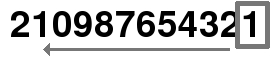• Calculate the sum of the numbers of odd and even positions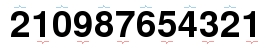• Odd : 1 + 3 + 5 + 7 + 9 + 1 = 26
• Even : 2 + 4 + 6 + 8 + 2 = 22

### EAN 13 checksum formula :

( 10 - [ (3 * Odd + Even) modulo 10 ] ) modulo 10

Thus, the example :

• (10 - [ (3 * 26 + 22) module 10 ] ) modulo 10
• (10 - [ (78 + 22) module 10 ] ) modulo 10
• (10 - [ (100) module 10 ] ) modulo 10
• (10 - [ 0 ] ) modulo 10
• (10 - 0) modulo 10
• 10 modulo 10
• 0

So the checksum of 210987654321 is 0

### EAN 13 checksum generator :

EAN 13 message : Checksum :

## Binary sequence of EAN 13 barcode

#### Start

A start sequence starts the barcode : 101

The 13 digits will be separated into 2 parts, namely the left part from the 1st au 7th digit and the right part from the 8th au 13th digit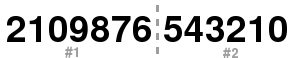#### Part #1

The first part is encoded according to the following table :

digitsequence
0000000
1001011
2001101
3001110
4010011
5011001
6011100
7010101
8010110
9011010

Thus for each bit of the first digit, a sequence of 6 "0" or "1" will be used to encode the 6 next digits according to the following tables :

digittable 0table 1
000011010100111
100110010110011
200100110011011
301111010100001
401000110011101
501100010111001
601011110000101
701110110010001
801101110001001
900010110010111

For example, the first digit is 2, the tables used for the digit 2 to 7 will be defined by the sequence 001101:

• The "1" will result from table "0"
• The "0" will result from table "0"
• The "9" will result from table "1"
• ...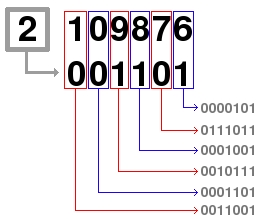Part #1 will be0011001 0001101 0010111 0001001 0111011 0000101

#### Intermediate

An intermediate sequence separate the 2 parts : 01010

#### Part #2

The second part is encoded according to the following table :

digittable 2
01110010
11100110
21101100
31000010
41011100
51001110
61010000
71000100
81001000
91110100

Part #2 will be 1001110 1011100 1000010 1101100 1100110 1110010

#### Stop

A stop sequence ends the barcode : 101

### Summary of the sequence of the EAN 13

• start : 101
• part #1
• center : 01010
• part #2
• stop : 101

## Graphical representation of the EAN 13 barcode

Each element of the binary sequence is represented by a space of equal width, it is a "1" or "0". The "1" are represented by spaces filled and "0" with empty spaces.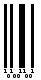## Online EAN 13 barcode generator

Please fill in the code :